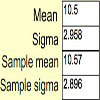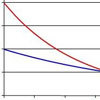#### You may also like### Spinners

How do scores on dice and factors of polynomials relate to each other?### Random Inequalities

Can you build a distribution with the maximum theoretical spread?### Into the Exponential Distribution

Get into the exponential distribution through an exploration of its pdf.

# Data Matching

### Why do this problem?

We hope that the intrigue of a mystery to be solved will spark students' curiosity and help them to appreciate the importance of being able to solve inverse problems, where you are given data and have to figure out the underlying structure.

This problem illustrates the concept of a probability distribution: various results are possible, and each result occurs with a certain probable frequency. It will help students to understand that even though each part of a random process is unpredictable, a large sample of data contains predictable patterns.

### Possible Approach

This activity works particularly well if students have access to computers or tablets so that they can use built-in statistical techniques. The data is presented in a GeoGebra worksheet, and is also available as a csv file.

Alternatively, you could print off Data Matching and cut out the cards for students to group.

Invite students to work in pairs to analyse the data sets, write down what they notice, draw any appropriate diagrams or work out summary statistics. Once they have grouped the sets, bring the class together to discuss the reasoning behind their grouping. Encourage them to present their arguments as clearly as possible. Do the others agree or disagree? Can the others refine their argument?

### Key Questions

Describe the cards in words.
How might you start to quantify the data on each card more precisely? How would you represent this?
Can you spot any patterns occurring in some of the cards? Does this help you to give a grouping?
Are there any graphs or diagrams you could draw to represent the distributions?

### Possible Extension

Once the cards are sorted, students could suggest the probability distributions from which the cards were drawn.

### Possible Support

The Stage 4 problem Which list is which? introduces a similar activity with just two data sets, so might be easier for students to get started on.